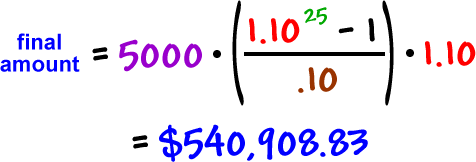Let's try something messier:

If we invest \$400 a month at 8.4%, how much will we have in 40 years?

investment amount = \$400

growth factor = \$1.007

interest per period = .007

number of periods = 480TRY IT:

Invest \$250 a month at 9%.  How much will you have in 20 years?

One more...  and this one we'll do yearly instead of monthly.  (So, we'll have to assume it's compounded yearly.)

If we invest \$5000 a year at 10%, how much will we have in 25 years?

investment amount = \$5000

growth factor = \$1.10

interest per period = .10

number of periods = 25